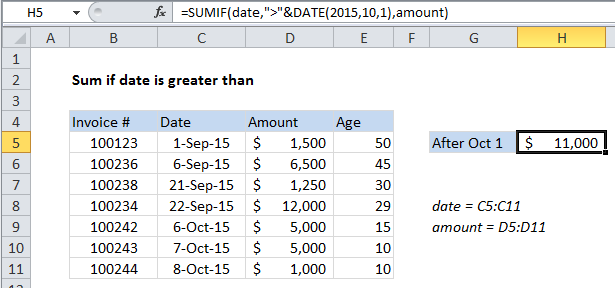## Excel Office

Excel How Tos, Tutorials, Tips & Tricks, Shortcuts

# Sum if date is greater than in Excel

This tutorial shows how to Sum if date is greater than in Excel using the example below;

## Formula

`=SUMIF(range,">"&DATE(year,month,day),sum_range)`## Explanation

To sum if based on dates greater than a certain date, you can use the SUMIF function.

In the example shown, cell H5 contains this formula:

`=SUMIF(date,">"&DATE(2015,10,1),amount)`

This formula sums the amounts in column D when a date in column C is greater than Oct 1, 2015.

Also See:   Sum if begins with in Excel

### How the formula works

The SUMIF function supports Excel’s logical operators (i.e. “=”,”>”,”>=”, etc.), so you can use thee as you like in your criteria.

In this case, we want to match dates greater than Oct 1, 2015, so we are using the greater than (>) operator with the DATE function to create a date:

`">"&DATE(2015,10,1)`

The DATE function is a safe way to create dates for function criteria, because it eliminates problems associated with regional date settings.

Also See:   Sum if ends with in Excel

Note that we need to enclose the greater than operator in double quotes and join it to the date with an ampersand (&).

### Date as cell reference

If you want to expose the date on the worksheet so that it can be easily changed, use this formula:

`=SUMIF(date,">"&A1,amount)`

Where A1 is a reference to a cell that contains a valid date.

Also See:   Two ways to sum time over 30 minutes in Excel

### Alternative with SUMIFS

You can also use the SUMIFS function. SUMIFS can handle multiple criteria, and the order of the arguments is different from SUMIF. The equivalent SUMIFS formula is:

`=SUMIFS(amount, date,">"&DATE(2015,10,1))`

Notice that the sum range always comes first in the SUMIFS function.